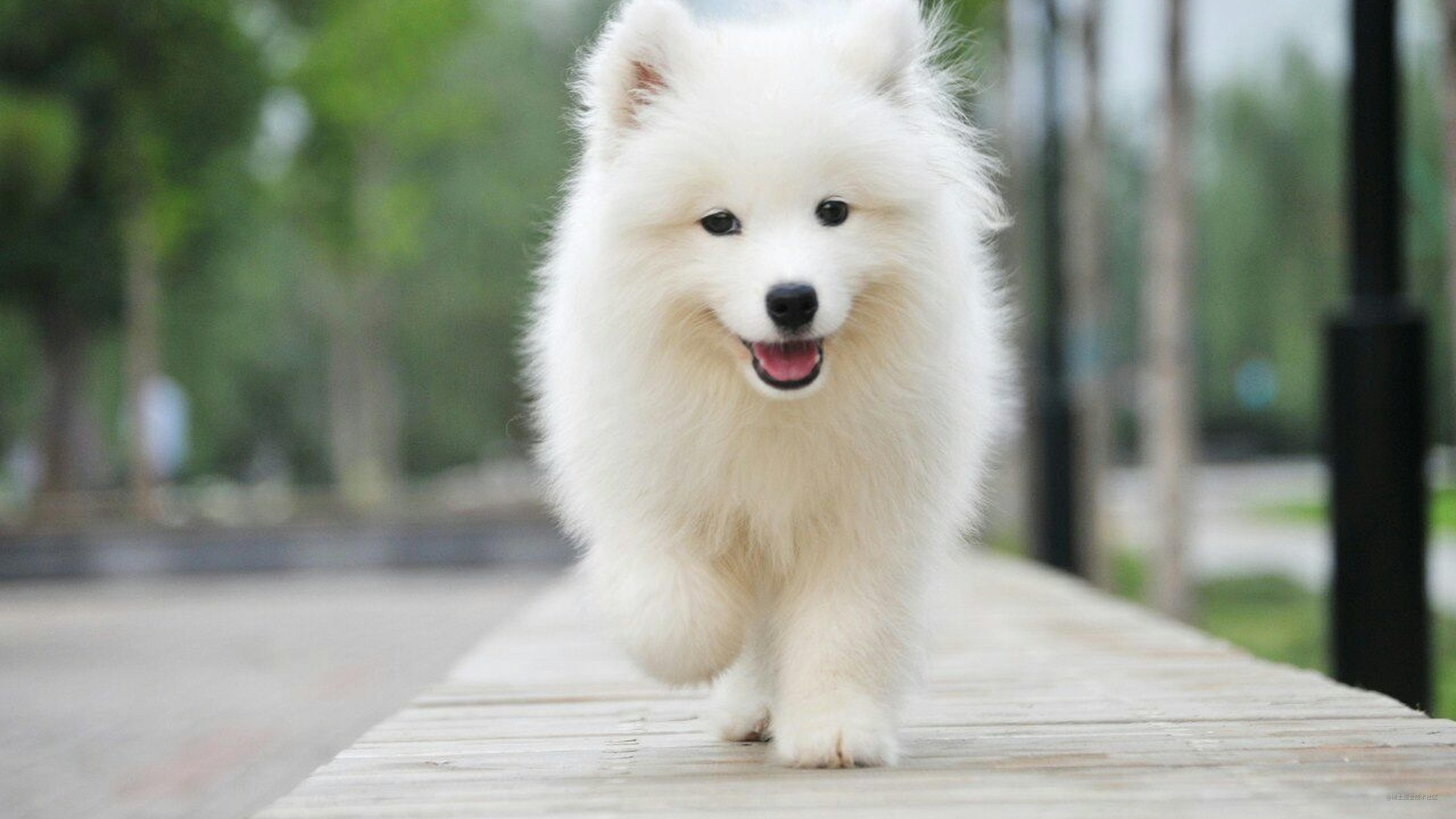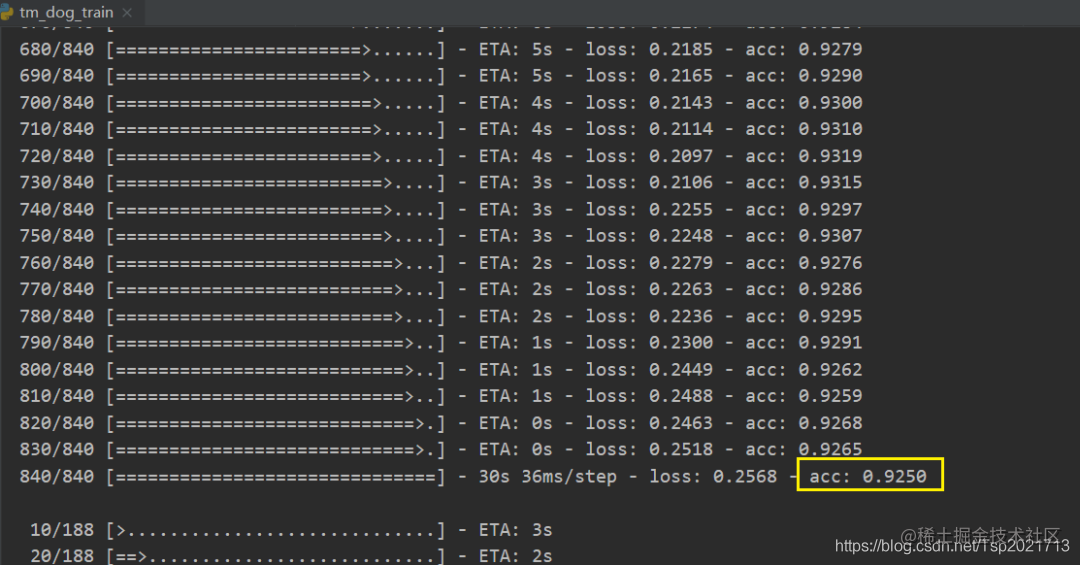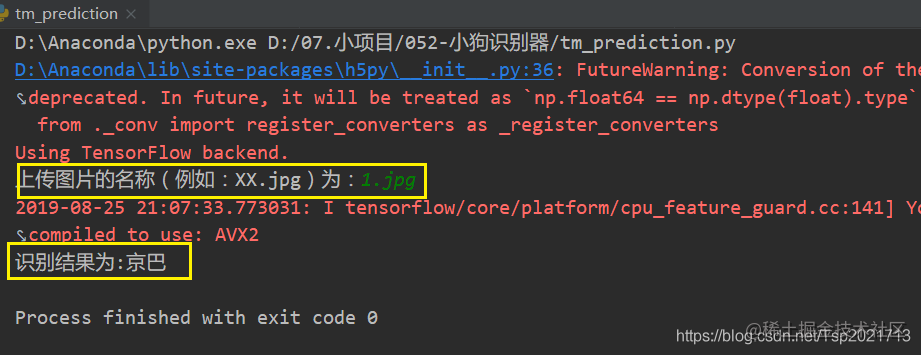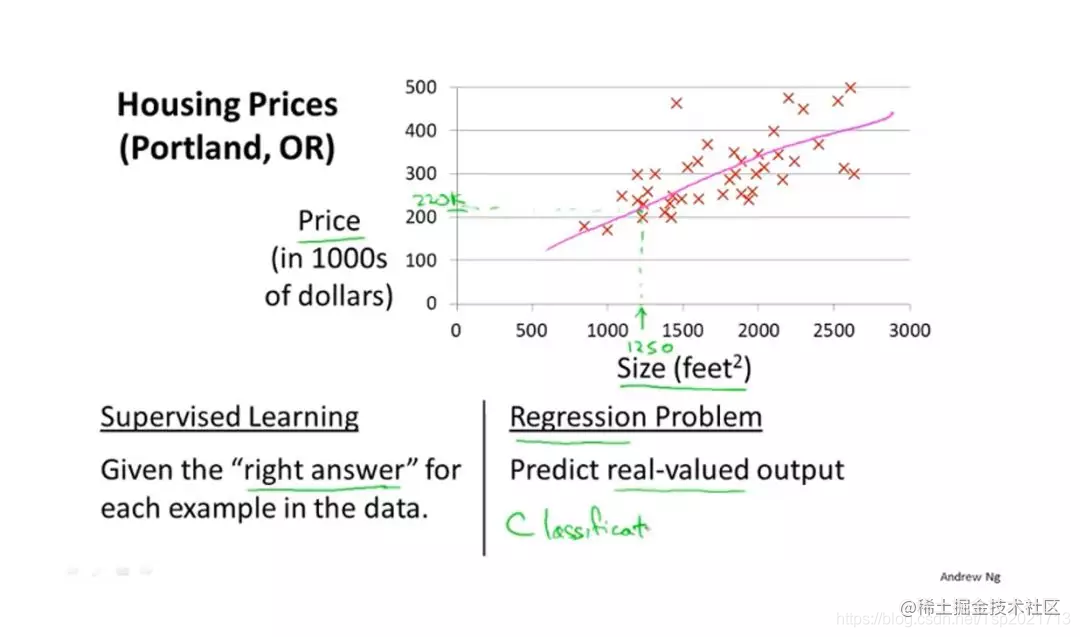# 用Python图像识别技术打造一个小狗分类器，实现让机器自己去“学习”~# 效果展示# 思路分析

• 1 准备数据集
• 2 数据集的预处理
• 3 搭建卷积神经网络
• 4 训练
• 5 预测

### 2 数据集的预处理

#### 1 统一尺寸为1001003（RGB彩色图像）

``````# 统一尺寸的核心代码
img = Image.open(img_path)
new_img = img.resize((100, 100), Image.BILINEAR)
new_img.save(os.path.join('./dog_kinds_after/' + dog_name, jpgfile))

#### 2 由于数据是自己下载的，需要制作标签（label），可提取图像名称的第一个数字作为类别。（重命名图片）

``````kind = 0

# 遍历京巴的文件夹
images = os.listdir(images_path)
for name in images:
image_path = images_path + '/'
os.rename(image_path + name, image_path + str(kind) +'_' + name.split('.')+'.jpg')

#### 3 划分数据集

840张图片做训练集，188张图片做测试集。

#### 4 把图片转换为网络需要的类型

``````# 只放了训练集的代码，测试集一样操作。
ima_train = os.listdir('./train')

# 图片其实就是一个矩阵（每一个像素都是0-255之间的数）（100*100*3）
# 1.把图片转换为矩阵
img = Image.open('./train/' + filename).convert('RGB')
return np.array(img)

x_train = []
# 2.把所有的图片矩阵放在一个列表里 (840, 100, 100, 3)
for i in ima_train:
x_train = np.array(x_train)
# 3.提取kind类别作为标签
y_train = []
for filename in ima_train:
y_train.append(int(filename.split('_')))

# 标签（0/1/2/3）(840,)
y_train = np.array(y_train)

# 我是因为重命名图片为（1/2/3/4），所以都减了1
# 为了能够转化为独热矩阵
y_train = y_train - 1

# 4.把标签转换为独热矩阵
# 将类别信息转换为独热码的形式（独热码有利于神经网络的训练）
y_train = np_utils.to_categorical(y_train)
y_test = np_utils.to_categorical(y_test)
print(y_test)

x_train = x_train.astype('float32')
x_test = x_test.astype('float32')

x_train /= 255
x_test /= 255
print(x_train.shape)  # (840, 100, 100, 3)
print(y_train.shape)  # (840,)

#### 3 搭建卷积神经网络

Keras是基于TensorFlow的深度学习库，是由纯Python编写而成的高层神经网络API，也仅支持Python开发。

``````# 1.搭建模型(类似于VGG，直接拿来用就行)
model = Sequential()
# 这里搭建的卷积层共有32个卷积核，卷积核大小为3*3，采用relu的激活方式。
# input_shape，字面意思就是输入数据的维度。

#这里使用序贯模型，比较容易理解
#序贯模型就像搭积木一样，将神经网络一层一层往上搭上去

model.add(Conv2D(32, (3, 3), activation='relu', input_shape=(100, 100, 3)))
model.add(Conv2D(32, (3, 3), activation='relu'))

model.add(Conv2D(64, (3, 3), activation='relu'))
model.add(Conv2D(64, (3, 3), activation='relu'))
#dropout层可以防止过拟合，每次有25%的数据将被抛弃

#### 4 训练``````# 编译模型
sgd = SGD(lr=0.01, decay=1e-6, momentum=0.9, nesterov=True)
model.compile(loss='categorical_crossentropy', optimizer=sgd, metrics=['accuracy'])

# 一共进行32轮
# 也就是说840张图片，每次训练10张，相当于一共训练84次
model.fit(x_train, y_train, batch_size=10, epochs=32)

# 保存权重文件（也就是相当于“房价问题的k和b两个参数”）
model.save_weights('./dog_weights.h5', overwrite=True)
# 评估模型
score = model.evaluate(x_test, y_test, batch_size=10)
print(score)

#### 5 预测

``````# 1.上传图片
name = input('上传图片的名称（例如：XX.jpg）为：')

# 2.预处理图片(代码省略)

# 3.加载权重文件

# 4.预测类别
classes = model.predict_classes(x_test)

target = ['京巴', '拉布拉多', '柯基', '泰迪']
# 3-泰迪 2-柯基 1-拉布拉多 0-京巴

# 5.打印结果
print("识别结果为:" + target[classes])

①　2000多本Python电子书（主流和经典的书籍应该都有了）

②　Python标准库资料（最全中文版）

③　项目源码（四五十个有趣且经典的练手项目及源码）

④　Python基础入门、爬虫、web开发、大数据分析方面的视频（适合小白学习）

⑤　Python学习路线图（告别不入流的学习）

All done～详见个人简介或者私信获取完整源代码。。

# 往期回顾

Python实现“假”数据

Python爬虫鲁迅先生《经典语录》

Python爬虫豆瓣热门话题